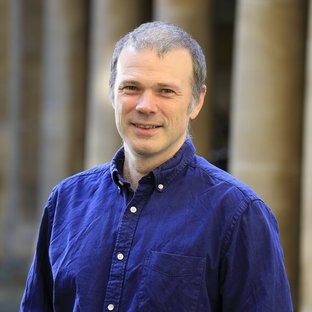# Professor Stuart White

Tutorial Fellow in Mathematics

## Biography

After my PhD at the University of Edinburgh, I spent a year at Texas A&M University as a postdoc, before returning to spend 12 years in Scotland at the University of Glasgow.  I joined St John’s College as a tutorial fellow in Mathematics in 2019.

For as long as I can remember, I’ve always enjoyed puzzles and games, and this led me naturally to mathematics.  Since then I’ve become attracted both by the beauty of mathematics, and the ‘ah ha’ moment when one finally understands how things fit together. Despite it’s abstract nature, mathematics is a very human activity; I really enjoy working on mathematics in a team, blending different prospectives and ideas.

## Teaching

I teach tutorials for a range of predominately pure mathematics courses in first and second year, and supervise DPhil students on topics related to my research interests.  Communication is key in university mathematics, where we’re not so interested in a number, or final answer, so much as the argument that gets us there. Tutorials are a great place for developing our mathematical communication skills; for me to understand how the students think and vice versa.

## Research Interests

My research focuses on operator algebras. This is a branch of functional analysis with connections to many other branches of pure mathematics. You can think of functional analysis as the place where linear algebra hits mathematical analysis; I’m interested in infinite dimensional vector spaces, which behave a little differently from the finite dimension spaces that we’ll primarily encounter in the first couple of years of the Mathematics degree.   One difference is that when we give an infinite dimensional vector space an appropriate notion of a distance, it’s no longer the case that all linear maps are automatically continuous.

The central objects I study are C*-algebras and von Neumann algebras. These are *-subalgebras of the bounded operators on a Hilbert space which are closed in the norm and weak operator topology respectively --- so in particular these algebras arise naturally in the theory of unitary representations of groups --- but they really best defined abstractly.  These classes of algebras have the flavour of topology and measure theory: abelian C* and von Neumann algebras are appropriate function algebras on locally compact Hausdorff and measure spaces respectively, while in the non-abelian setting ideas from algebraic topology pervade the theory of C*-algebras and von Neumann algebras provide a framework for the study of non-commutative probability.  In my own work, I’m particular interested in the interplay and transfer of ideas between C* and von Neumann algebras, and how this leads to classification results